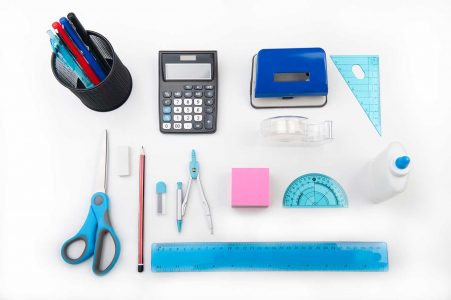An increase in class workload stresses up students as they often have to do more work to achieve good grades than they can handle. In turn they get frustrated and are unable to focus and think straight. Students will overschedule their plans to meet up with the class workload in other to get good grades but eventually because these tasks are too much for them, they end up messing everything up. We understand that each student has many courses to deal with these days and each course demands the pupil’s full attention. The student does not have enough time to worry about remembering minor formulae and searching for equations to handle simple or complex math problems. This is why we at Square Footage Area have decide to design solutions for your everyday math problems.

We are in the era of technology and as a result of that human life, in every aspect, is adapting to these changes in order to suit our present environment. These changes do not only affect humans but also institutions. The introduction of information technology and smart phones in our lives has done a lot of good for schools and students. Many courses can be signed up to online. So we thought a solution to our student’s maths problems should also be made available in a medium they often use in the form of online tools.Here are the calculators we have designed for you and the problems you can solve using them:

Area of a Circular Border
The area of a circle is the space contained within its circumference (outer perimeter). To find out the area of a circle border, we need to know its inner diameter and the width of the circular border. The diameter and border width should be measured in feet (ft) for square footage calculations and if needed, converted to inches (in), yards (yd), centimetres (cm), millimetres (mm) and metres (m).

#### Formula:

Outer diameter = Inner diameter + (2 x Border width)
Outer area = π x (Outer diameter/2)^2
Inner area = π x (Inner diameter/2)^2
Area of a Circle Border = Outer area – Inner area
π = 3.142
Answer = (Outer area – Inner area) square area
Abbreviations of unit area: ft2, in2, yd2, cm2, mm2, m2

Area of a Rectangular Border
The area of a rectangle is the space contained within a set of lines. To find out the area of a rectangle border, we need to subtract the area of the inner rectangle from the area of the outer rectangle. These lines should be measured in feet (ft) for square footage calculations and if needed, converted to inches (in), yards (yd), centimetres (cm), millimetres (mm) and metres (m).

#### Formula:

Inner area = (Length x Width)
Total area = (Length + (2 x Width of border)) x (Width + (2 x Width of border))
Area of a Rectangle border = Total area – Inner area
Answer = (Side length x Side length) square area
Abbreviations of unit area: ft2, in2, yd2, cm2, mm2, m2

Area of A Square
The area of a square is the space contained within a set of lines. These lines should be measured in feet (ft) for square footage calculations and if needed, converted to inches (in), yards (yd), centimetres (cm), millimetres (mm) and metres (m).

#### Formula:

Area of a Square= Side length x Side length
Answer = (Length x length) square area
Abbreviations of unit area: ft2, in2, yd2, cm2, mm2, m2

Area of a Rectangle
The area of a square is the space contained within a set of parallel lines – each set of different lengths. These lines should be measured in feet (ft) for square footage calculations and if needed, converted to inches (in), yards (yd), centimetres (cm), millimetres (mm) and metres (m).

#### Formula:

Area of a Square= Side length x Side width
Answer = (Length x Width) square area
Abbreviations of unit area: ft2, in2, yd2, cm2, mm2, m2Area of a Triangle
The area of a triangle is the space contained within its 3 sides. To find out the area of a triangle, we need to know the length of its three sides. The sides should be measured in feet (ft) for square footage calculations and if needed, converted to inches (in), yards (yd), centimetres (cm), millimetres (mm) and metres (m).

#### Formula:

Area of a Triangle = (1/4) x √ [ (a+b+c) x (b+c-a) x (c+a-b) x (a+b-c) ]
Answer = ((1/4) x √ [ (a+b+c) x (b+c-a) x (c+a-b) x (a+b-c) ])
Abbreviations of unit area: ft2, in2, yd2, cm2, mm2, m2

Area of a Circle
The area of a circle is the space contained within its circumference (outer perimeter). To find out the area of a circle, we need to know its diameter which is the length of its widest part. The diameter should be measured in feet (ft) for square footage calculations and if needed, converted to inches (in), yards (yd), centimetres (cm), millimetres (mm) and metres (m).

#### Formula:

Area of a Circle = π x (Diameter/2)2
π = 3.142
Answer = (π x (Diameter/2)2) square area
Abbreviations of unit area: ft2, in2, yd2, cm2, mm2, m2

Area of a Trapezoid
To find out the area of a trapezoid, we need to know the length of two parallel sides and the distance (height) between them. The sides should be measured in feet (ft) for square footage calculations and if needed, converted to inches (in), yards (yd), centimetres (cm), millimetres (mm) and metres (m).

#### Formula:

Area of a Trapezoid = ((a + b) / 2) h
Answer = (((a + b) / 2) h) square area
Abbreviations of unit area: ft2, in2, yd2, cm2, mm2, m2

Want more calculators? Let us know which calculators you need and we will try our best to add them to our website. Write to us.